Next: 2.2.3 Calculation of physical Up: 2.2 Mapping to a Previous: 2.2.1 Principles of the

## 2.2.2 Equivalence to a resonant level model

Now we will compare this system of differential equations with the flow equations for a resonant level model (RLM). This will lead to the key result of this chapter: the RLM can be used as an effective model for the complicated strong-coupling Kondo model.

The Hamiltonian of the resonant level model is given by(2.8)

Following the same flow equation approach as previously in the SIKM, we establish a solution to the RLM (2.8). A detailed description of the flow equation solution can be found in Ref. (20). One finds the following flow equations for the parameters in (2.8)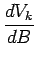(2.9)(2.10)(2.11)

It should be noted that this yields the exact analytical solution. Having established the flow equations to solve both the SIKM and the RLM, respectively, one can now show an approximate equivalence of these two models. We introduce the substitution(2.12)

and notice that with this substitution the two set of flow equations (2.5,2.6) and (2.9-2.11) become equivalent for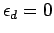in the RLM, with the exception of the logarithmic term in (2.5). Thus we now have established an approximate mapping of the SIKM onto the RLM by means of (2.12) in the sense that their flow equation diagonalization is identical. We shall refer to this relation by introducing the effective hybridization function: the RLM with this non-trivial hybridization function can be used as an effective model for the SIKM in the Kondo limit (small coupling limit)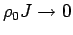. Since this noninteracting RLM is a simple, quadratic Hamiltonian, this mapping will allow us to read off and understand many properties of the complicated many-body Kondo physics in an intuitive and straightforward way. It will turn out that the deviations offrom a constant hybridization function encode the quasiparticle interaction and therefore the many-body Kondo physics in this quadratic effective Hamiltonian.

Notice that the above mapping between the SIKM and the RLM becomes exact at the Toulouse point (21)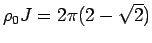since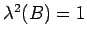for all flow parameters. One easily verifies that the effective RLM then has a constant hybridization function,. In this case, our mapping just reduces to the observation already made by Toulouse that the partition function of the Kondo model for this specific coupling constant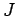is exactly equivalent to the partition function of a quadratic Hamiltonian.(21)

In order to specify the functionin the Kondo limit it is best to not directly use relation (2.12), but to determine the effective hybridization function from matching a correlation function in the SIKM and the RLM. We have chosen the-correlation function, evaluated it with respect to (2.4) for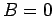, and then chosein the RLM such that this coincided with the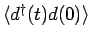-correlation function. The resultingagrees with (2.12) in the high- and low-energy regimes, with deviations only in the crossover region. However, the mapping from the Kondo model to the effective RLM becomes better since this procedure manages to partly also take the logarithmic term in (2.5) into account.

The resulting effective hybridization function can be scaled into a dimensionless form with one dimensionful parameter(2.13)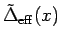is a universal function in the Kondo limit (). It is depicted in Fig. 2.1 for, and coincides with its universal form for(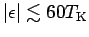, i.e. this should be sufficient for most practical purposes(22)). For larger energies the effective hybridization function begins to cross over into linear behavior with logarithmic corrections depending on the bare coupling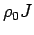.The following function provides an excellent fit (see Fig. 2.1)(2.14)

with the parameters from Table 2.1.

Table: Result of the fit (2.14) to the effective hybridization.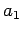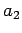0.829 0.536 0.00324

A similar analysis based on the comparison of flow equations shows that the above mapping between the SIKM and the noninteracting RLM can be extended to the case of a Kondo Hamiltonian (2.1) with a nonvanishing local magnetic field(2.15)

by settingin the RLM. However, the mapping with the above effective hybridization function becomes less accurate fordue to the approximate nature of the flow equation solution (2.5-2.7). We will discuss this point in more detail below.

Summing up, as long as we are interested in static quantities in a local magnetic field smaller than approximatelyand/or dynamical correlation functions for energies smaller than approximately, we can use the RLM with the effective hybridization function (2.14) to describe the physics of the SIKM in the small coupling limit. The only undetermined parameter in the RLM is the energy scalethat explicitly depends on. This overall energy scale is proportional to. Notice that the non-perturbative behavior of this energy scale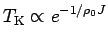(2.16)

follows correctly from the original flow equations (2.5-2.7), compare Ref. (16).Next: 2.2.3 Calculation of physical Up: 2.2 Mapping to a Previous: 2.2.1 Principles of the
© Cyrill Slezak